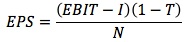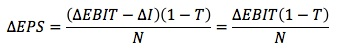### Why should I choose AnalystNotes?

Simply put: AnalystNotes offers the best value and the best product available to help you pass your exams.

##### Subject 2. Financial Risk and Financial Leverage
Financial risk is the additional risk placed on the common stockholders as a result of the decision to use fixed-income securities (debt and preferred stock). Increases in financial leverage (the use of fixed-income securities) increases financial risk and the expected return of stockholders, due to the obligation of servicing the fixed interest payments.

The questions are: Is the increased rate of return sufficient to compensate shareholders for the increased risk? What is the optimal financial structure to maximize stock price and the firm's value?

Financial risk depends on two factors:

• Cash flow volatility. The more volatile (stable) a firm's cash flows, the higher (lower) the financial risk.
• Financial leverage. The higher the financial leverage, the higher the financial risk.

As a general proposition, financial leverage raises the expected rate of return, but at the cost of increased financial risk (and thus total risk). So, you are faced with a trade-off: if you use more financial leverage, you increase the expected rate of return, which is good, but you also increase risk, which is bad.

The degree of financial leverage (DFL) measures the financial risk.It shows how a given percentage change in EBIT per share will affect EPS.

The equation above is developed as follows:where:
I = interest paid
T = marginal tax rate
N = number of shares outstanding

I is a constant so ΔI = 0, therefore:Now the percentage change in EPS is the change in EPS divided by the original EPS, which is:DFL is defined as the percentage change in earnings per share (EPS) divided by the percentage change in EBIT.Consider a company with EBIT = \$100,000 and interest = \$20,000. Its DFL = 100,000 / (100,000 - 20,000) = 1.25. Therefore, a 100% increase in EBIT would result in a 125% increase in EPS.

Unlike operating leverage, the degree of financial leverage is most often a choice by the company's management. Companies with a higher ratio of tangible assets to total assets may have higher degrees of financial leverage because lenders may feel more secure that their claims would be satisfied in the event of a downturn.